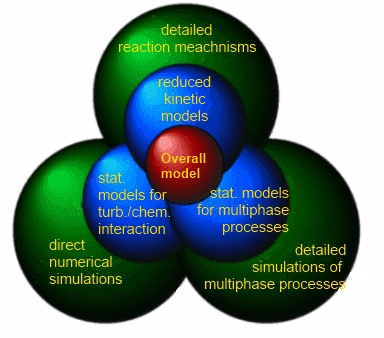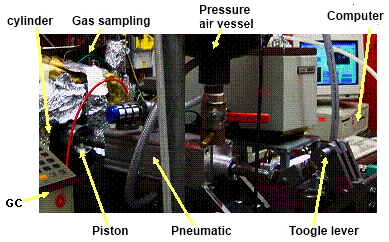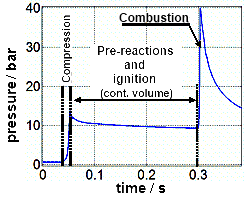# Reduced description of the chemistry-turbulence interaction

Turbulent flames are characterised by a broad spectrum of time, length and velocity scales.

A direct solution of the conservation equations for momentum, energy, mass and particle masses is possible in principle, but as demonstrated above quite impracticable. Moreover, the high parametric sensitivity of turbulent processes means that for practical relevant quantities, such as e.g. the mean temperature and mean pollutant concentrations, averaging over many such direct numerical simulations would be necessary. However, due to the high non-linearity of the chemical kinetics, a ‘naïve’ calculation of the mean chemical source terms from the means of the temperature and of species concentrations leads to totally incorrect results. For this reason, simulations of technical combustion systems are performed using statistical models that rely on a consideration of the joint probability density function (PDF) for velocities and scalars. It turns out, however, that it is very difficult to determine the (space- and time-dependent) PDF. The Institute of Technical Thermodynamics uses a very accurate but also very demanding method for determining the PDF, based on the solution of a transport equation. In this method, the chemical kinetics are treated exactly. The turbulent mixing processes, however, must be modelled. In addition to the problem of modelling the molecular transport processes, the high dimensionality of the transport equation hampers its solution even further, since on top of the velocity components each chemical species goes into the transport equation as an independent variable. Therefore, Monte Carlo solution methods are the usual technique being relied upon.

Reduced description of multiphase processes

Many technical combustion processes rely on liquid fuels (e.g. diesel engines). This poses an additional difficulty for mathematical modelling, since multiphase processes are involved. The complexity then depends crucially on the fuel spray’s properties. A statistical formulation is obtained by considering the probability density function for the spray, i.e. for the disperse phase. In analogy with the PDF transport equation for the gas phase, the underlying spray equation can be solved with Monte Carlo methods. It is, however, difficult to determine the exchange terms between the disperse liquid phase and the gas phase, which are affected by evaporation velocity, the fine structure of the reaction zone around the flame and possibly even by processes inside the droplet (convection, heat conduction). Here too, detailed simulations allow close insight into the coupling of the flow, transport and reaction processes (see above).

The previous sections described, using the example of chemical kinetics, droplet combustion and turbulence-chemistry interaction, how reduced models for chemical kinetics and the chemistry-turbulence interaction can be derived from detailed models and detailed simulations. In order to simulate technical combustion processes, these part-models must be linked so as to form an overall model that permits efficient simulation of the entire process. This is shown schematically in Fig. 6.Schematic diagram of the hierarchical modelling strategy

#### Experimental investigation of combustion processes

Even though numerical simulations are becoming ever more precise, powerful, and thanks to the constant increase in computers’ performance, also ever more practicable, the empirical investigation of combustion processes remains indispensable. Experiments are performed in order to verify the predictions of the computational models, in order to obtain data about unknown quantities in the computational models, and last but not least, in order to discover fundamentally new phenomena. The applications range from the determination of thermodynamic quantities through the measurement of ignition delays in fuel/air mixtures to laser diagnostic investigation of combustion in engines and in turbulent flames.

The nature of the combustion process imposes stringent demands on the measurement techniques. Very high temperatures and pressures are reached (2000 °C is regularly exceeded, pressures above one hundred bar occur in diesel engines). The instrumentation needs to be reliable and accurate even under such extreme conditions. Furthermore, very rapid temporal transients often occur during the technically highly important process of turbulent combustion; the temperature at a test point may rise by 1000 °C within fractions of a millisecond. A good measurement method should be able to resolve these time-scales. Similar demands apply also to spatial resolution: in many combustion processes, the relevant scales lie in the submillimetre range, and the measurement method should be capable of resolving regions of this size. Another particularity results from the great sensitivity of many combustion systems to external interference: given a small modification of the external conditions (which may derive from the measuring instrument itself), the system’s behaviour can change significantly. The measurement method should, therefore, be non-intrusive.

It is clear that any one method on its own is unlikely to satisfy this broad range of requirements. There exist several methods, which can be used singly or in combination depending on the precise requirements. In principle, we can distinguish between fundamental experiments and experiments on real systems. The former are used largely independently of concrete technical systems or technical specifications, for the development and validation of models or for fundamental research. Accordingly, the results are of general interest and are applied in the long term in very different technical systems. The latter are mostly linked to concrete technical developments, and are used in experimental set-ups that are identical with real systems or are very similar to them. Typically, the knowledge gained thereby is specific to the particular system being investigated, and its application mostly takes place in the short term.

#### Validation experiment for chemical kinetics

The question of the exact numerical values of certain parameters included in combustion modelling, is a focal point of the research. For example, the reaction process illustrated in Fig. 1 includes numerical parameters for every individual elementary reaction, namely the so-called Arrhenius parameters, in particular the activation energy of this reaction. Often, accurate knowledge of such parameters is decisive for the accuracy and predictive power of the modelling’s outcomes. For many technically important fuels, however, the Arrhenius parameters are known only very approximately. Moreover, often it is not clear whether a reaction flowchart includes all the essential elementary reactions. In order to be able to estimate the flowchart’s accuracy, experiments are performed on a system, whose behaviour given the availability of a reaction flowchart can be calculated in advance simply and with high accuracy. One such system is the rapid compression machine (RCM), used at the Institute of Technical Thermodynamics to measure ignition delays of fuel-air mixtures. The RCM is a piston-cylinder device, similar to a reciprocating engine. The piston is pushed pneumatically into the cylinder within about one hundredth of a second, as a result of which the cylinder’s charge is compressed quasi-adiabatically and thus reaches very high temperatures (above 1000 K). The piston is locked mechanically at the upper dead-centre position, such that the volume remains constant over time following compression.Photograph of the rapid compression maschine at the Institute of Technical Thermodynamics Typical cylinder pressure curve in the RCM. The first pressure increase (at approx. 0.05 s) results from the compression of the gas by the piston, the second (0.3 s) occurs after self-ignition of the gas. The pressure drop after compression and after combustion is the result of heat losses in the gas at the cylinder’s walls.

In this experiment, the fuel/air mixture to be investigated is introduced into the cylinder (in the gas phase), compressed and then the time until the onset of self-ignition measured. This time can be compared with the separately calculated ignition delay, from which predications can be made about the accuracy of the underlying reaction flowchart and possible improvements to it.

Start | Previous | Page: 1 - 2 - 3 - 4 - 5 - 6 | Next | End